# Practical exercise 1: AROUND THE SUN

The Earth moves around the Sun in an almost perfect circular orbit5 with radius a = 1 au and with a period T = 365,25 dní.

a) From the 3rd Kepler's law, determine the mass of the Sun in kilograms. For comparison, determine how many times it is more massive than the Earth (the mass of the Earth is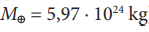)

b) Assuming that the Earth moves in a precise circular orbit around the Sun, calculate the speed of the Earth in its orbit.

Solution:

a) First we convert the units of quantities into the SI system: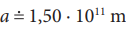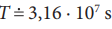. It remains to be substituted into the 3rd Kepler's law: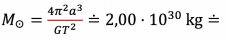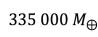The actual mass of the Sun is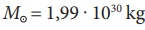,  so the value calculated by us is correct.

b) According to the assumption, the trajectory of the Earth is a circle, i.e. the Earth´s orbit is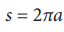, in 1 year, therefore the orbital velocity is equal to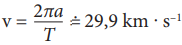.

The Earth´s orbit around the Sun is not an exact circle and its speed varies between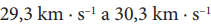.